# Continuous function

(diff) ← Older revision | Latest revision (diff) | Newer revision → (diff)
This page lists a core term of calculus. The term is used widely, and a thorough understanding of its definition is critical.
See a complete list of core terminology

## Definition for functions of one variable

### At a point

Consider a function$f$ and a real number$c$ such that$f$ is defined in an open interval containing$c$, i.e.,$f$ is defined at$c$ and on the immediate left and right of$c$. We say that$f$ is continuous at$c$ if it satisfies the following equivalent definitions:

No. Shorthand What the definition says
1 in terms of limits$\lim_{x \to c} f(x) = f(c)$. In words, the limit of$f(x)$ as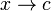$x \to c$ exists and equals the value of the function at$c$.
2 in terms of one-sided limits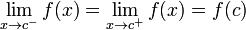$\lim_{x \to c^-} f(x) = \lim_{x \to c^+} f(x) = f(c)$. In words, the left hand limit of$f$ at$c$, the right hand limit of$f$ at$c$, and the value of$f$ at$c$ are all equal.
3 in terms of left and right continuity$f$ is both left and right continuous at$c$.
4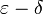$\varepsilon-\delta$ For every$\varepsilon > 0$, there exists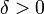$\delta > 0$ such that for all$x$ satisfying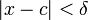$|x - c| < \delta$ (i.e.,$x \in (c - \delta,c + \delta)$), we have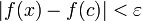$|f(x) - f(c)| < \varepsilon$ (i.e.,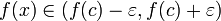$f(x) \in (f(c) - \varepsilon,f(c) + \varepsilon)$).
4'$\varepsilon-\delta$ (variant) For every$\varepsilon > 0$, there exists$\delta > 0$ such that for all$x$ satisfying$0 < |x - c| < \delta$ (i.e.,$x \in (c - \delta,c + \delta) \setminus \{ c \}$), we have$|f(x) - f(c)| < \varepsilon$ (i.e.,$f(x) \in (f(c) - \varepsilon,f(c) + \varepsilon)$).
5 in terms of centered open balls (same as$\varepsilon-\delta$ without the symbols) For every open ball (i.e., open interval) centered at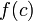$f(c)$, there is an open ball (i.e., open interval) centered at$c$ such that the image of the open ball centered at$c$ lies inside the open ball centered at$f(c)$. [SHOW MORE]
6 in terms of not necessarily centered open balls For every open ball (i.e., open interval) containing$f(c)$, there is an open ball containing$c$ such that the image of the open ball containing$c$ lies inside the open ball containing$f(c)$.

### Definition of one-sided continuity

Left continuity: Consider a function$f$ and a real number$c$ such that$f$ is defined at$c$ and on the immediate left of$c$. We say that$f$ is left continuous at$c$ if the left hand limit of$f$ at$c$ exists and equals$f(c)$, i.e.,$\lim_{x \to c^-} f(x) = f(c)$.

Right continuity: Consider a function$f$ and a real number$c$ such that$f$ is defined at$c$ and on the immediate right of$c$. We say that$f$ is right continuous at$c$ if the right hand limit of$f$ at$c$ exists and equals$f(c)$, i.e.,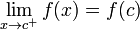$\lim_{x \to c^+} f(x) = f(c)$.

### On an interval

Consider an interval, which may be open or closed at either end, and may stretch to$-\infty$ on the left or$\infty$ on the right. A function from such an interval to the real numbers is termed continuous if it satisfies the following two conditions:

1. It is continuous (in the sense of continuous at a point) at all points in the interior of the interval, i.e., all points such that there is an open ball containing the point lying inside the domain interval.
2. It has the appropriate one-sided continuity at endpoints: If the interval has a left endpoint (e.g., the interval is of the form$[a,b]$,$[a,b)$, or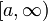$[a,\infty)$, then it must be right continuous at the left endpoint ($a$ in all three example intervals). If the interval has a right endpoint (e.g., the interval is of the form$[a,b]$,$(a,b]$, or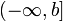$(-\infty,b]$), then it must be left continuous at the right endpoint ($b$ in all three example intervals).